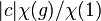Degree of irreducible representation divides order of group

This article gives the statement, and possibly proof, of a constraint on numerical invariants that can be associated with a finite group

This article states a result of the form that one natural number divides another. Specifically, the (degree of a linear representation) of a/an/the (irreducible linear representation) divides the (order) of a/an/the (group).
View other divisor relations |View congruence conditions
This fact is related to: linear representation theory
View other facts related to linear representation theoryView terms related to linear representation theory |

Statement

Let$G$ be a finite group and$\varphi$ an irreducible representation of$G$ over an algebraically closed field of characteristic zero (or, more generally, over any splitting field of characteristic zero for$G$). Then, the degree of$\varphi$ divides the order of$G$.

Related facts

Other facts about degrees of irreducible representations

Further information: Degrees of irreducible representations

Breakdown for a field that is not algebraically closed

Let$G$ be the cyclic group of order three and$\R$ be the field. Then, there are two irreducible representations of$G$ over$\R$: the trivial representation, and a two-dimensional representation given by the action by rotation by multiples of$2\pi/3$. The two-dimensional representation has degree$2$, and this does not divide the order of the group, which is$3$.

We still have the following results:

Facts used

The table below lists key facts used directly and explicitly in the proof. Fact numbers as used in the table may be referenced in the proof. This table need not list facts used indirectly, i.e., facts that are used to prove these facts, and it need not list facts used implicitly through assumptions embedded in the choice of terminology and language.
Fact no. Statement Steps in the proof where it is used Qualitative description of how it is used What does it rely on? Other applications
1 Character orthogonality theorem: The part relevant for us is: for an irreducible representation over a splitting field of characteristic zero with character$\chi$,$\sum_{g \in G} \chi(g)\overline{\chi(g)} = |G|$ Step (1) Equation setup that we then tinker with. click here
2 Size-degree-weighted characters are algebraic integers: This states that for an irreducible linear representation$\varphi$ of a finite group$G$ over an algebraically closed field of characteristic zero (or more generally, over any splitting field), with character$\chi$, a conjugacy class$c$ in$G$ and an element$g \in c$, the number$|c|\chi(g)/\chi(1)$ (with$1$ denoting the identity element of the group) is an algebraic integer. Step (3) Show certain parts of an expression are algebraic integers. algebraic number theory + linear representation theory click here
3 Characters are algebraic integers Step (4) Show certain parts of an expression are algebraic integers. basic linear representation theory click here

Proof

This proof uses a tabular format for presentation. Provide feedback on tabular proof formats in a survey (opens in new window/tab) | Learn more about tabular proof formats|View all pages on facts with proofs in tabular format

Given: A finite group$G$, an irreducible linear representation$\varphi$ of$G$ over a splitting field of characteristic zero for$G$, with character$\chi$ and degree$d$. Note that$d$ equals$\chi(1)$, i.e., the value of$\chi$ at the identity element of$G$.

To prove:$d$ divides the order of$G$.

Proof:

Step no. Assertion/construction Facts used Given data used Previous steps used Explanation
1 The following holds:$\sum_c |c| \chi(c) \overline{\chi(c)} = |G|$ where the sum is over all conjugacy classes$c$ of$G$, and where$\chi(c)$ denotes the value of$\chi$ at any element of$c$. Fact (1)$\varphi$ is irreducible over a splitting field of characteristic zero, with character$\chi$. Follows from fact (1). The$|c|$ comes because for each conjugacy class$c$,$|c|$ elements of the class appear in the full statement of the column orthogonality theorem.
2$\sum_c |c|\chi(c) \overline{\chi(c)}/\chi(1) = |G|/d$ Step (1) Divide both sides of step (1) by$d = \chi(1)$.
3 Each$|c|\chi(c)/\chi(1)$ is an algebraic integer for each conjugacy class$c$. Fact (2)$\varphi$ is irreducible over a splitting field of characteristic zero, with character$\chi$.
4 Each$\overline{\chi(c)}$ is an algebraic integer for each conjugacy class$c$. Fact (3)$\chi$ is a character. The complex conjugate of an algebraic integer is also an algebraic integer.
5$\sum_c |c|\chi(c) \overline{\chi(c)}/\chi(1)$ is an algebraic integer. Steps (3), (4) The set of algebraic integers forms a ring, so a finite sum of products of algebraic integers is an algebraic integer.
6$|G|/d$ is an algebraic integer. Steps (2), (5) By Step (5), the left side of Step (2) is an algebraic integer, hence so is the right side.
7$|G|/d$ is a positive integer, so$d$ divides$|G|$. Step (6) Both$|G|$ and$d$ are positive integers, hence their quotient is a positive rational number. The only way a rational number can be an algebraic integer is if it is an integer, hence the conclusion.